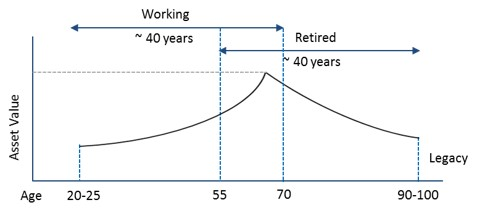# Asset Growth Using Rule of 72

## Achieving a multi-million dollar asset value in one’s lifetime is more possible and likely than you may think it is. It can be achieved by committing capital to a long-term growth vehicle that, thanks to the power of compounding, provides a consistently increasing return!

Rule of 72 is an elegant way to quantify investment return from compound growth. It states that the value doubles every ‘N’ years when the underlying investment grows at ‘R%’ annually, provided N x R is 72.

For example, a conservative portfolio returning 4% CAGR (Compound Annual Growth Rate) will double every 18 years (where, 18 x 4 is 72). Likewise, an aggressive portfolio returning 8% CAGR will double every 9 years. Rule of 72 can also be applied for non-investment scenarios; for example, prices in a 2% low inflationary environment will double every 36 years. Thus, over a lifetime, one will see prices go up 4x because of inflation.

Let us now apply the concept of Rule of 72 to an asset growth achieved over the life of a person. We all, typically, start working in our early 20s and work for about 35-40 years before retiring. We then also need to plan for as much as another 35-40 years in retirement, due to the continued increase in life expectancy.Using the Rule of 72, we can see that an investment amount doubles every decade when growing at 7.2% CAGR. This expected return may be a bit on the aggressive side, but it helps to emphasize the power of compound growth in a person’s lifetime. Let’s say a working professional manages to save \$250K by age 35. Just that portion of their wealth alone can potentially grow to \$8M by the time they reach age 85.Of course, we have simplified the assumptions here with regard to both the consistency of growth rate and money staying invested. We have not considered the impact of taxes and inflation on asset growth, which we delve into in “Effect of Taxes vs. Inflation on Asset Growth” article. However, it is not hard to understand, however, how just one segment of wealth can grow into a large sum within an investor’s lifetime, precisely as our example illustrates. Committing capital to a long-term growth vehicle that provides consistent return, even if it is conservative, is one of the best ways to build assets.

A video presentation of this blog is available at “Asset Growth Using Rule of 72.”

Math behind Rule of 72
As discussed in the “Power of Compounding” article, the compound interest formula is, Future Value (FV) = Present Value (PV) x (1+R)N. If the value doubles, then FV/PV is 2.  Apply natural logarithm on both sides, N x ln(1+R) = 69.3. Apply Taylor’s series approximation to simplify left hand side of the equation to N x R, and adjust the constant value on the right hand side to 72, since 72 has many multiples. The resulting equation is N x R = 72.

We specialize in tax-free retirement strategy and investments such as IUL, Annuity and LTC. Prefer a quick and complimentary consultation? Just email us at Karthik@FinCrafters.com